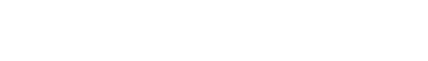Press "Enter" to skip to content

### 2. 算法模型#### 需要注意的部分是#### 预测公式：#### 实验验证### 3. 代码复现

```import numpy as np
import pandas as pd
import tensorflow as tf
from tensorflow import keras
from sklearn.model_selection import train_test_split```

#### 定义模型

```class AutoRec(keras.Model):
def __init__(self, feature_nums, hidden_units, **kwargs):
super(AutoRec, self).__init__()
self.feature_nums = feature_nums # 基于物品则为物品特征数-即用户数，基于用户则为物品数量
self.hidden_units = hidden_units # 论文中的k参数
self.encoder = keras.layers.Dense(self.hidden_units, input_shape=[self.feature_nums], activation='sigmoid') # g映射
self.decoder = keras.layers.Dense(self.feature_nums, input_shape=[self.hidden_units]) # f映射
def call(self, X):
# 前向传播
h = self.encoder(X)
y_hat = self.decoder(h)
return y_hat```

#### 定义损失函数

`mask_zero` 表示没有评分的部分不进入损失函数，同时要保证数据类型统一 `tf.int32,tf.float32` 否则会报错。

```class  Mse_Reg(keras.losses.Loss):
def __init__(self, model, reg_factor=None):
super(Mse_Reg, self).__init__()
self.model = model
self.reg_factor = reg_factor

def call(self, y_true, y_pred) :
y_sub = y_true - y_pred
mask_zero = y_true != 0
mask_zero = tf.cast(mask_zero, dtype=y_sub.dtype)
y_sub *= mask_zero
mse = tf.math.reduce_sum(tf.math.square(y_sub)) # mse损失部分
reg = 0.0
if self.reg_factor is not None:
weight = self.model.weights
for w in weight:
if 'bias' not in w.name:
reg += tf.reduce_sum(tf.square(w)) # 求矩阵的Frobenius范数的平方
return mse + self.reg_factor * 0.5 * reg
return mse```

#### 定义RMSE评价指标

```class RMSE(keras.metrics.Metric):
def __init__(self):
super(RMSE, self).__init__()
self.res = self.add_weight(name='res', dtype=tf.float32, initializer=tf.zeros_initializer())
def update_state(self, y_true, y_pred, sample_weight=None):
y_sub = y_true - y_pred
mask_zero = y_true != 0
mask_zero = tf.cast(mask_zero, dtype=y_sub.dtype)
y_sub *= mask_zero
values = tf.math.sqrt(tf.reduce_mean(tf.square(y_sub)))
self.res.assign_add(values)
def result(self):
return self.res```

#### 定义数据集

`get_data` 表示从path中加载数据，然后加数据通过pandas的透视表功能构造一个行为物品，列为用户的矩阵；

`data_iter` 表示通过 `tf.data` 构造数据集。

```# 定义数据
def get_data(path, base_items=True):
data = pd.read_csv(path)
rate_matrix = pd.pivot_table(data, values='rating', index='movieId', columns='userId',fill_value=0.0)
if base_items:
return rate_matrix
else :
return rate_matrix.T

def data_iter(df, shuffle=True, batch_szie=32, training=False) :
df = df.copy()
X = df.values.astype(np.float32)
ds = tf.data.Dataset.from_tensor_slices((X, X)).batch(batch_szie)
if training:
ds = ds.repeat()
return ds```

#### 训练模型

```path = 'ratings.csv' # 我这里用的是10w数据，不是原始的movielens-1m
# I-AutoRec，num_users为特征维度
rate_matrix = get_data(path)
num_items, num_users = rate_matrix.shape
# 划分训练测试集
BARCH = 128
train, test = train_test_split(rate_matrix, test_size=0.1)
train, val = train_test_split(train, test_size=0.1)
train_ds = data_iter(train, batch_szie=BARCH, training=True)
val_ds = data_iter(val, shuffle=False)
test_ds = data_iter(test, shuffle=False)
# 定义模型
net = AutoRec(feature_nums=num_users, hidden_units=500) # I-AutoRec, k=500
net.compile(loss=Mse_Reg(net), #keras.losses.MeanSquaredError(),
optimizer=keras.optimizers.Adam(),
metrics=[RMSE()])
net.fit(train_ds, validation_data=val_ds, epochs=10, validation_steps=2, steps_per_epoch=train.shape//BARCH)
loss, rmse = net.evaluate(test_ds)
print('loss: ', loss, ' rmse: ', rmse)```

#### 预测

```df = test.copy()
X = df.values.astype(np.float32)
ds = tf.data.Dataset.from_tensor_slices(X) # 这里没有第二个X了
ds = ds.batch(32)
pred = net.predict(ds)
# 随便提出来一个测试集中有的评分看看预测的分数是否正常,pred包含原始为0.0的分数现在已经预测出来分数的。
print('valid: pred user1 for item1: ', pred[X.argmax()], 'real: ', X[X.argmax()])```### 4. 小结

I-AutoRec推荐过程，需要输入物品的矩阵然后得到每个用户对物品的预测评分，然后取用户自己评分的Top可以进行推荐，U-AutoRec只需要输入一次目标用户的向量就可以重建用户对所有物品的评分，然后得到推荐列表，但是用户向量可能稀疏性比较大影响最终的推荐效果。

AutoRec使用了单层网络，存在表达能力不足的问题，但对于基于机器学习的矩阵分解，协同过滤来说，由于这层网络的加入特征的表达能力得到提高。